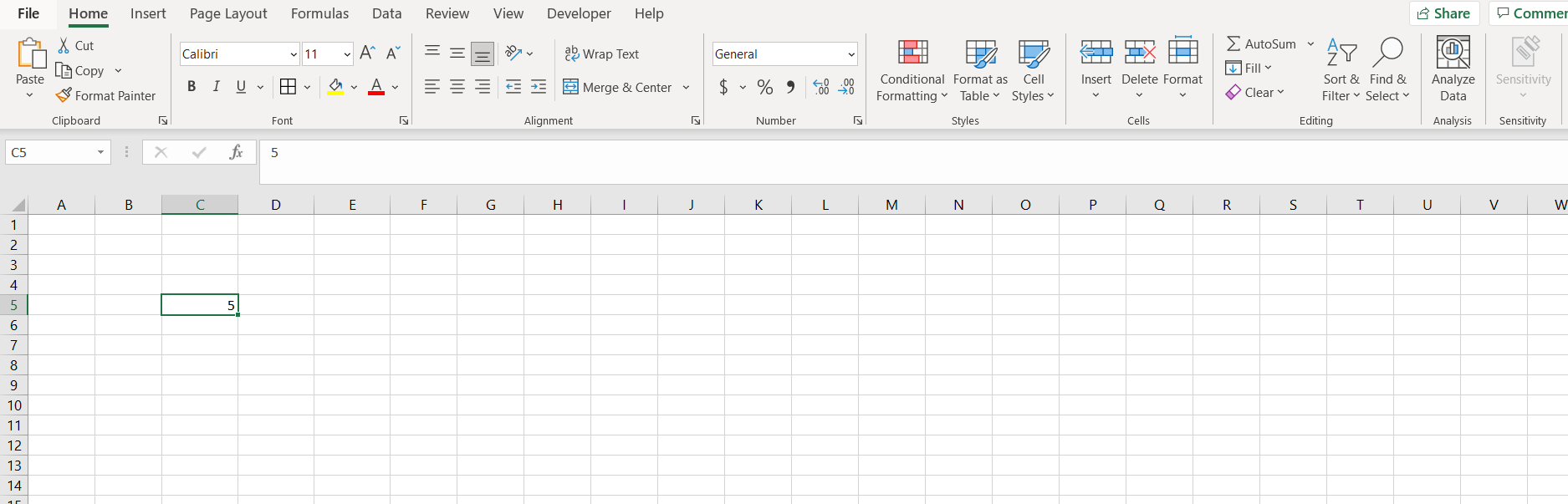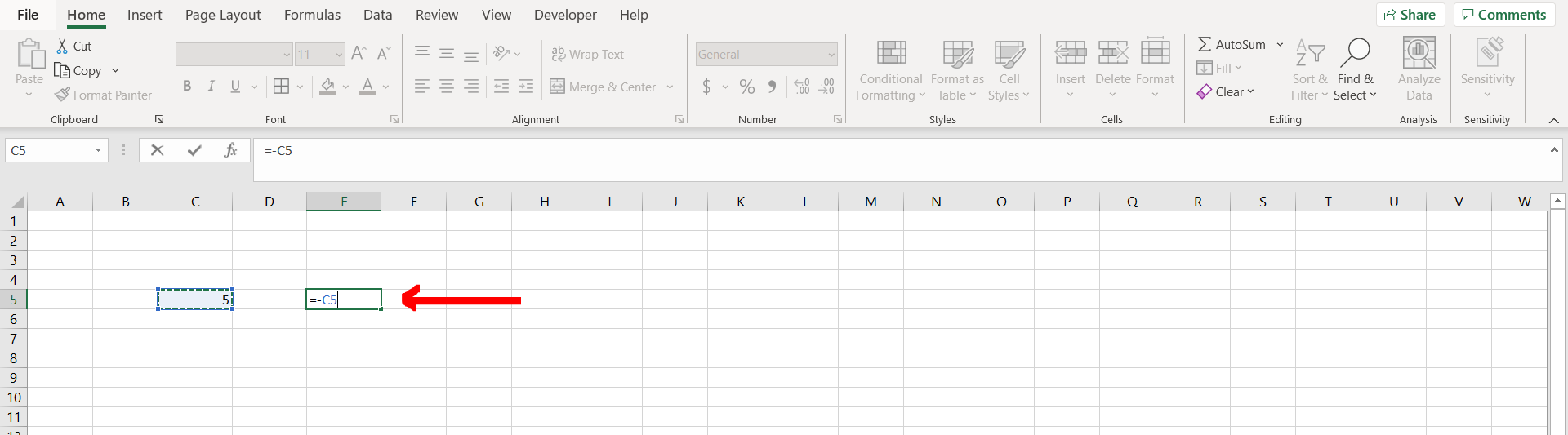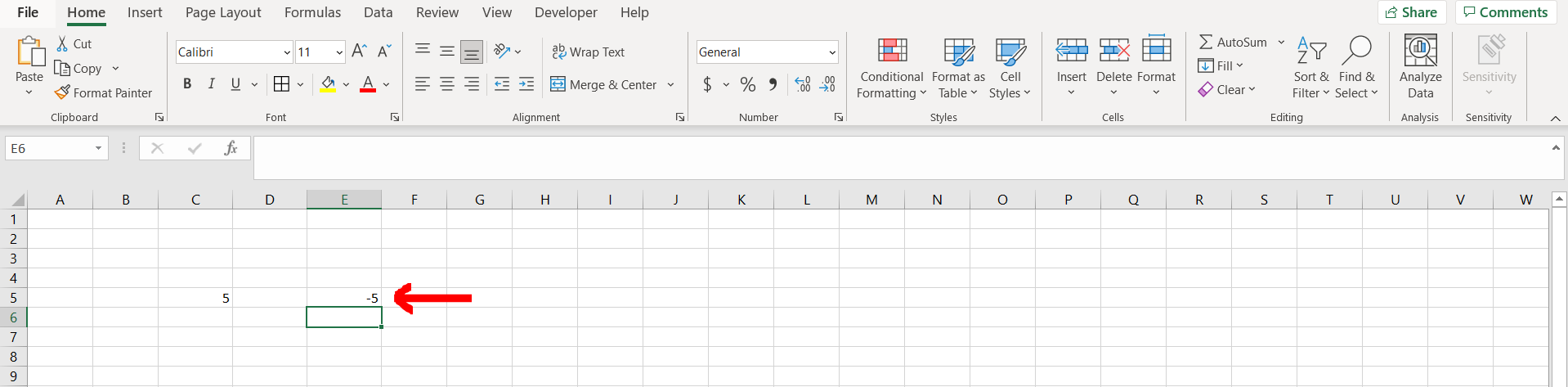# How to make a number negative in ExcelMicrosoft Excel offers a very interesting way to make a number negative. We can cater to this problem statement by using the “-” operator. We can perform the below mentioned way to make a number negative in excel:

### Step 1 – Excel sheet with a positive number– Open the desired Excel workbook containing a positive number which can be turned negative

### Step 2 – Typing the formula– Now type “=-”, and then reference to the cell containing the number as shown in the image above, and then hit Enter

### Step 3 – Number turned into negative– We can see that the number has been turned into negative